# 3天破9亿！上万条评论解读《西虹市首富》是否值得一看tomato = pd.DataFrame(columns=['date','score','city','comment','nick'])
for i in range(0, 1000):
j = random.randint(1,1000)
print(str(i)+' '+str(j))
try:
time.sleep(2)
html = requests.get(url=url).content
for item in data:
tomato = tomato.append({'date':item['time'].split(' '),'city':item['cityName'],
'score':item['score'],'comment':item['content'],
'nick':item['nick']},ignore_index=True)
tomato.to_csv('西虹市首富4.csv',index=False)
except:
continue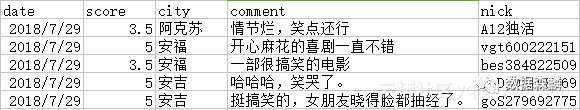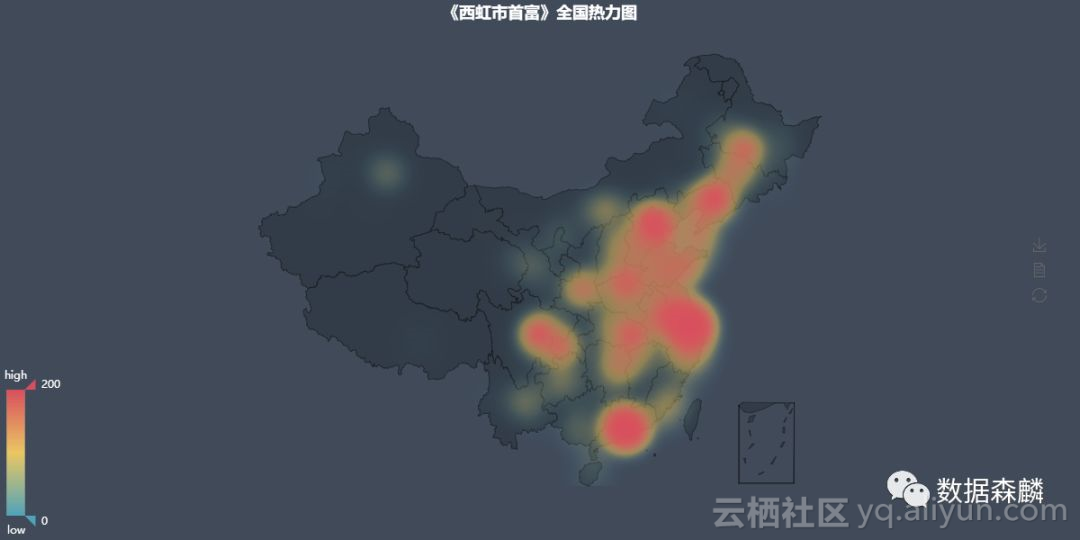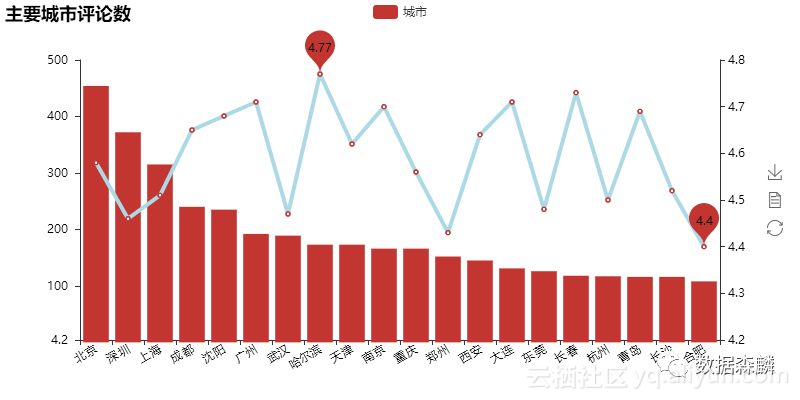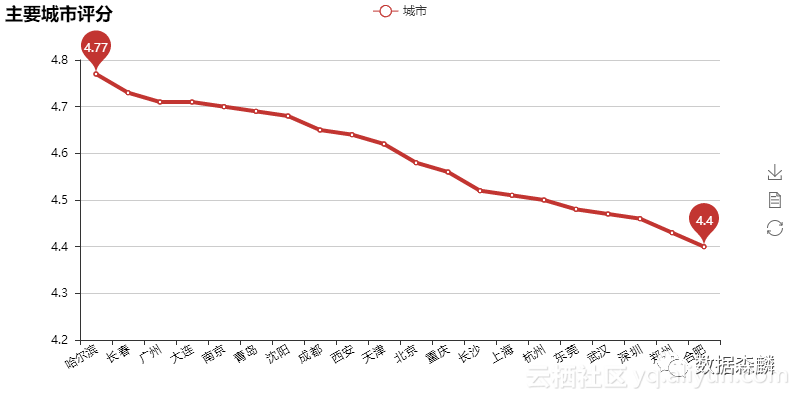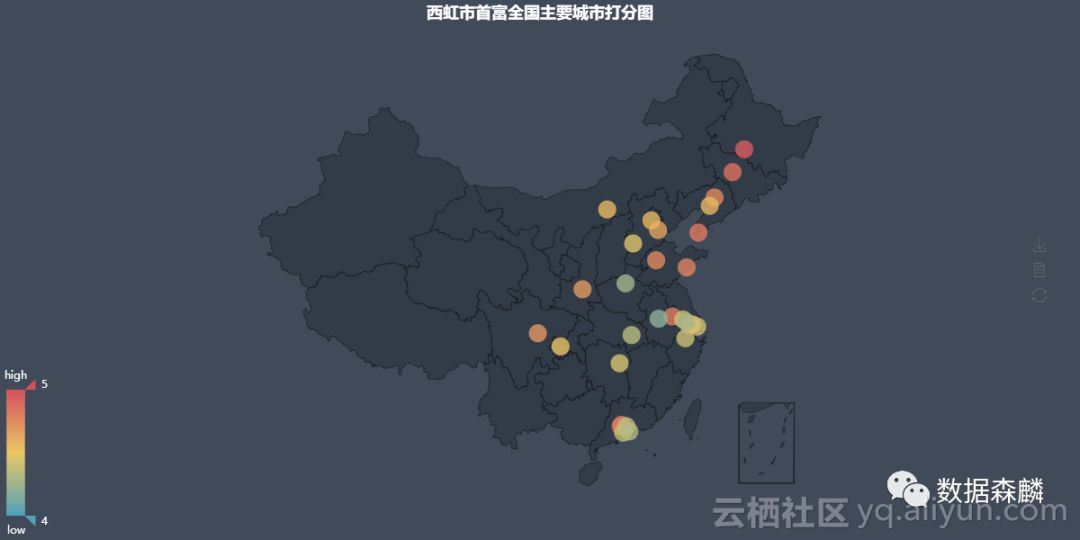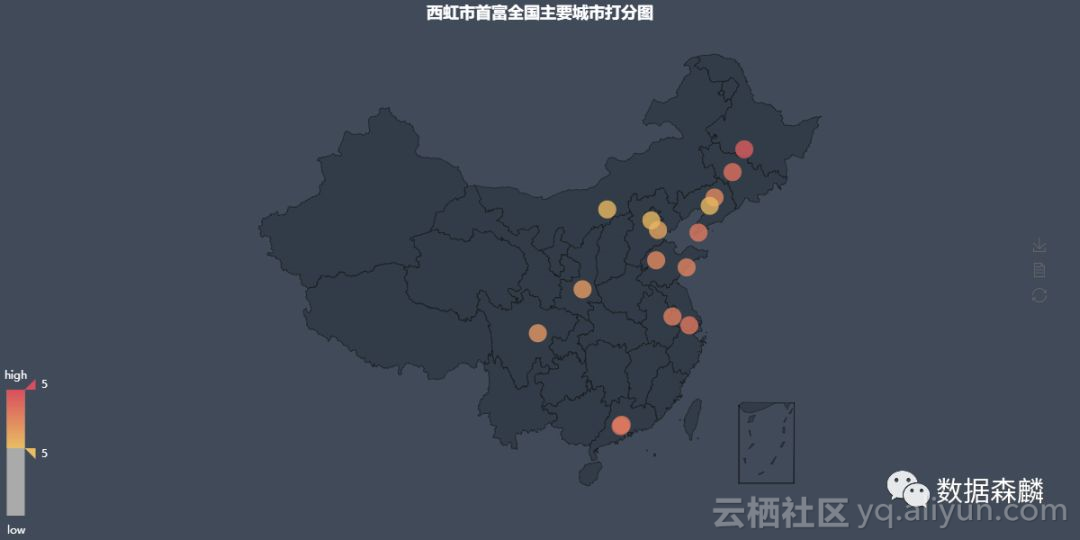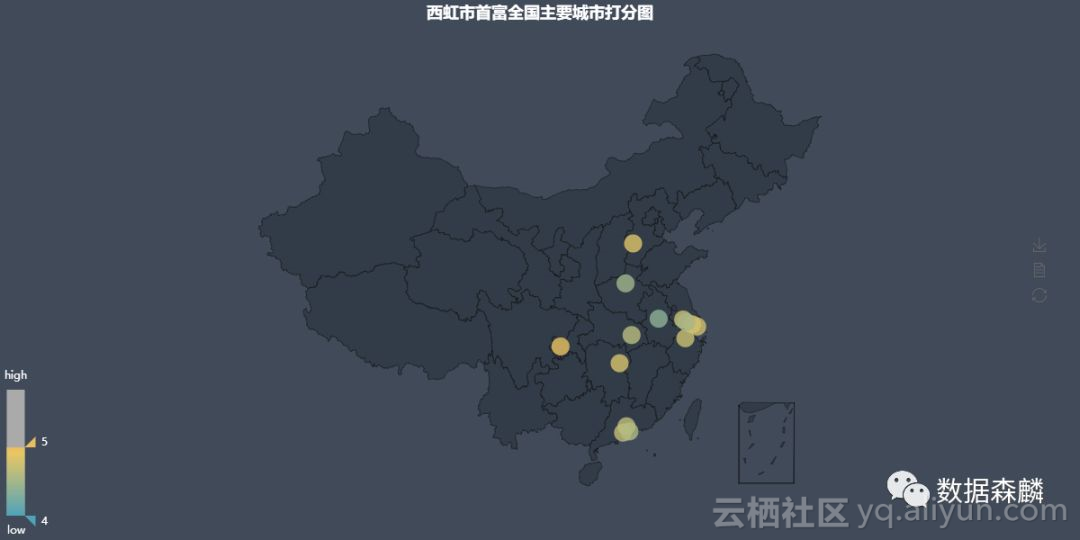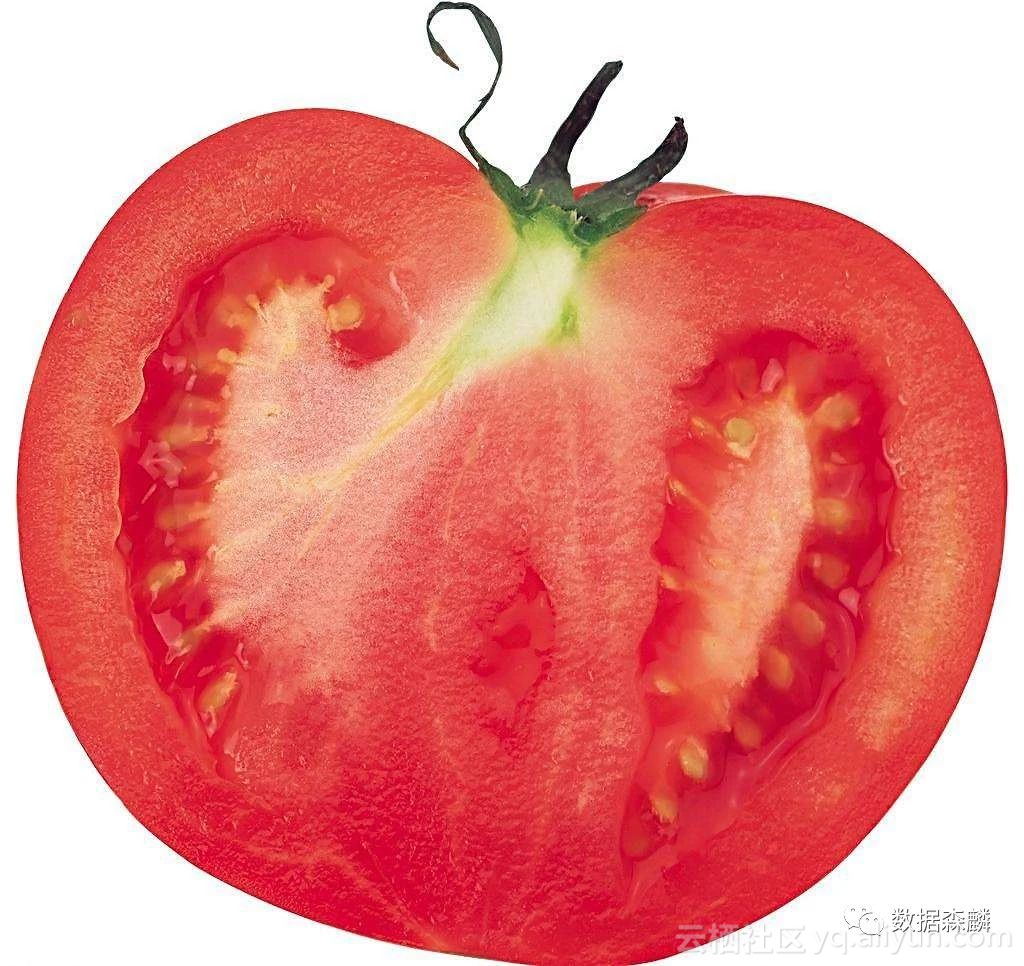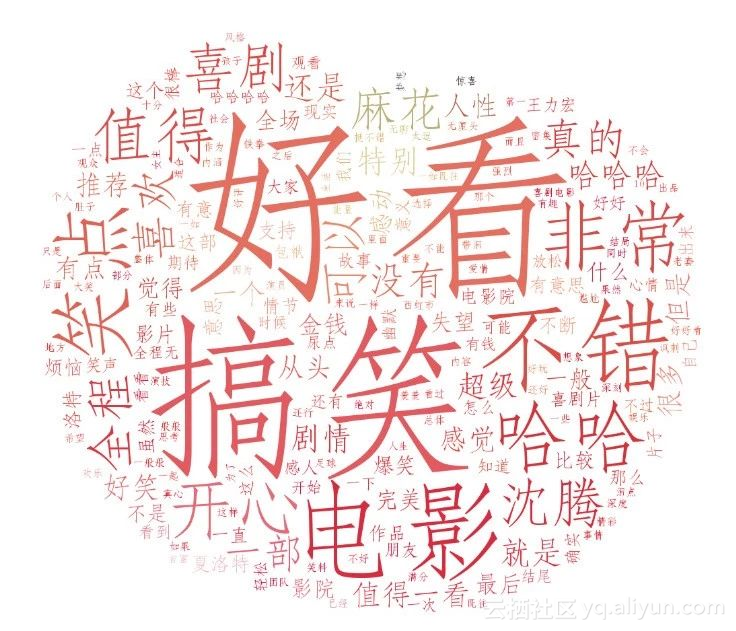tomato_com = pd.read_excel('西虹市首富.xlsx')
grouped=tomato_com.groupby(['city'])
grouped_pct=grouped['score'] #tip_pct列
city_com = grouped_pct.agg(['mean','count'])
city_com.reset_index(inplace=True)
city_com['mean'] = round(city_com['mean'],2)

data=[(city_com['city'][i],city_com['count'][i]) for i in range(0,city_com.shape)]
geo = Geo('《西虹市首富》全国热力图', title_color="#fff",
title_pos="center", width=1200,
height=600, background_color='#404a59')
attr, value = geo.cast(data)
geo.add("", attr, value, type="heatmap", visual_range=[0, 200],visual_text_color="#fff",
symbol_size=10, is_visualmap=True,is_roam=False)
geo.render('西虹市首富全国热力图.html')


city_main = city_com.sort_values('count',ascending=False)[0:20]
attr = city_main['city']
v1=city_main['count']
v2=city_main['mean']
line = Line("主要城市评分")
xaxis_rotate=30,yaxis_min=4.2,
mark_point=['min','max'],xaxis_interval=0,
line_color='lightblue',
line_width=4,mark_point_textcolor='black',
mark_point_color='lightblue',
is_splitline_show=False)
bar = Bar("主要城市评论数")
xaxis_interval =0,is_splitline_show=False)
overlap = Overlap()
# 默认不新增 x y 轴，并且 x y 轴的索引都为 0
overlap.render('主要城市评论数_平均分.html')


tomato_str =  ' '.join(tomato_com['comment'])
words_list = []
word_generator = jieba.cut_for_search(tomato_str)
for word in word_generator:
words_list.append(word)
words_list = [k for k in words_list if len(k)>1]
wc = WordCloud(background_color='white',  # 背景颜色
max_words=200,  # 最大词数
max_font_size=300,  # 显示字体的最大值
font_path="C:/Windows/Fonts/STFANGSO.ttf",
random_state=42,  # 为每个词返回一个PIL颜色
# width=1000,  # 图片的宽
# height=860  #图片的长
)
tomato_count = Counter(words_list)
wc.generate_from_frequencies(tomato_count)
# 基于彩色图像生成相应彩色
image_colors = ImageColorGenerator(back_color)
# 绘制词云
plt.figure()
plt.imshow(wc.recolor(color_func=image_colors))
plt.axis('off')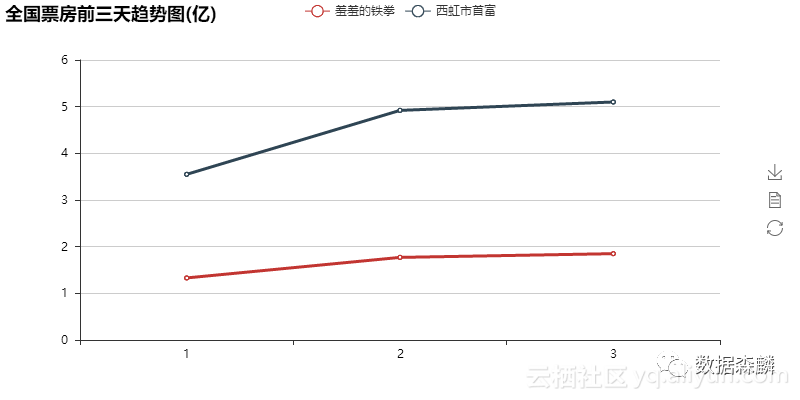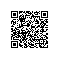Python爱好者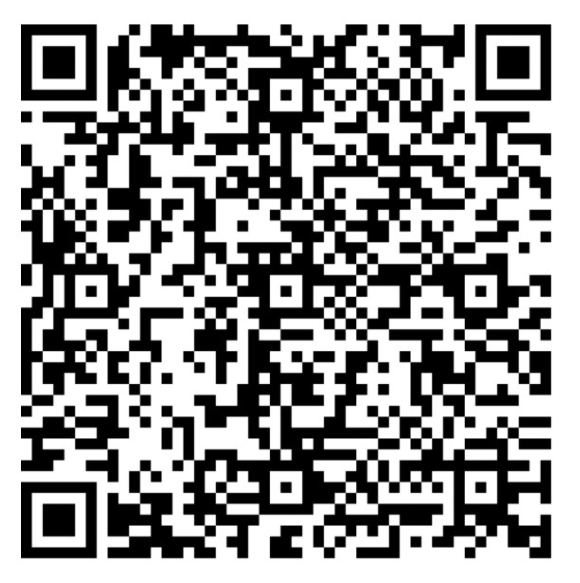使用钉钉扫一扫加入圈子
+ 订阅